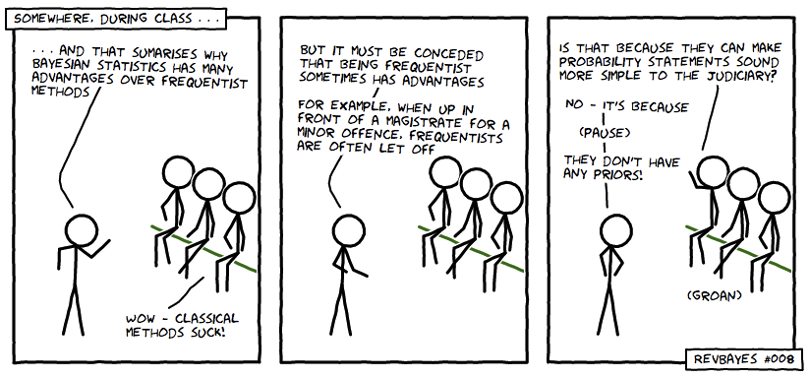# Why am I frequently Bayesian?

Dr. Juha Kreula, Senior Data Scientist
Data ScienceTo solve problems in the real world, one should choose the most appropriate approaches and tools available that are practical to use and hopefully general enough to productise. That said, a Bayesian toolbox can bring benefits by having a consistent framework to model unknown quantities, incorporate prior information, and, depending on the inference engine used, diagnose model fit to data.

In addition to such general considerations, I frequently find myself enjoying building and solving Bayesian statistical models. There is a lot of freedom and responsibility for the scientist to make sensible modelling choices.

Depending on the problem, these choices can include e.g. whether to use hierarchical/multilevel models, what the appropriate number of hierarchy levels is, which effects to set as population level and which as group level effects, and which probability distributions to use for the observation model, priors, hyperpriors, and so on.

Once the (first) model is defined, one must then solve the posterior distributions of its parameters (or rather, the posteriors are learned from the data). For learning, one can choose for example from sampling-based Markov chain Monte Carlo methods or from approaches based on approximating complex probability distributions with simpler ones, such as (automatic differentiation) variational inference techniques or integrated nested Laplace approximations (if working with latent Gaussian models and if posterior marginals suffice).

After model fitting, there come e.g. posterior predictive checks and other model validation and selection tools that help guide whether you had made sensible choices in the first place to explain the data. These mathematical and numerical techniques used are a big part of the fun of the Bayesian world to me.

To do the above Bayesian modelling steps, there are numerous advanced libraries, such as Stan, PyMC3, and R-INLA among others. While these mathematically and computationally sophisticated libraries can be used as black box tools, peeking under their hood can be a rewarding learning experience. So can be studying research articles and textbooks on Bayesian machine learning.

If you love applied math, numerical methods, and building statistical models, the versatility of the Bayesian way (when applicable) can provide a gratifying statistical machine learning toolbox for practical problems.

Share blog post